# NCERT Exemplar Solutions for Class 7 Maths Chapter 11 Exponents and Powers

NCERT Exemplar Solutions for Class 7 Maths Chapter 11 Exponents and Powers is the most preferred study material due to its unique description of the concepts. In Chapter 11, a distinctive attempt is made to build an understanding of the concepts. Pursuing this Chapter would ensure that you develop a piece of in-depth knowledge about the NCERT Exemplar Solutions for Class 7 Maths.

Exponents are the product of rational numbers multiplied several times by themselves. In this Chapter students are going to learn some important concepts which are as follows

• Laws of Exponents
• Multiplying Powers With The Same Base
• Dividing Powers With The Same Base
• Multiplying Powers With The Same Exponents
• Dividing Powers With The Same Exponents

## Download the PDF of NCERT Exemplar Solutions for Class 7 Maths Chapter 11 Exponents and Powers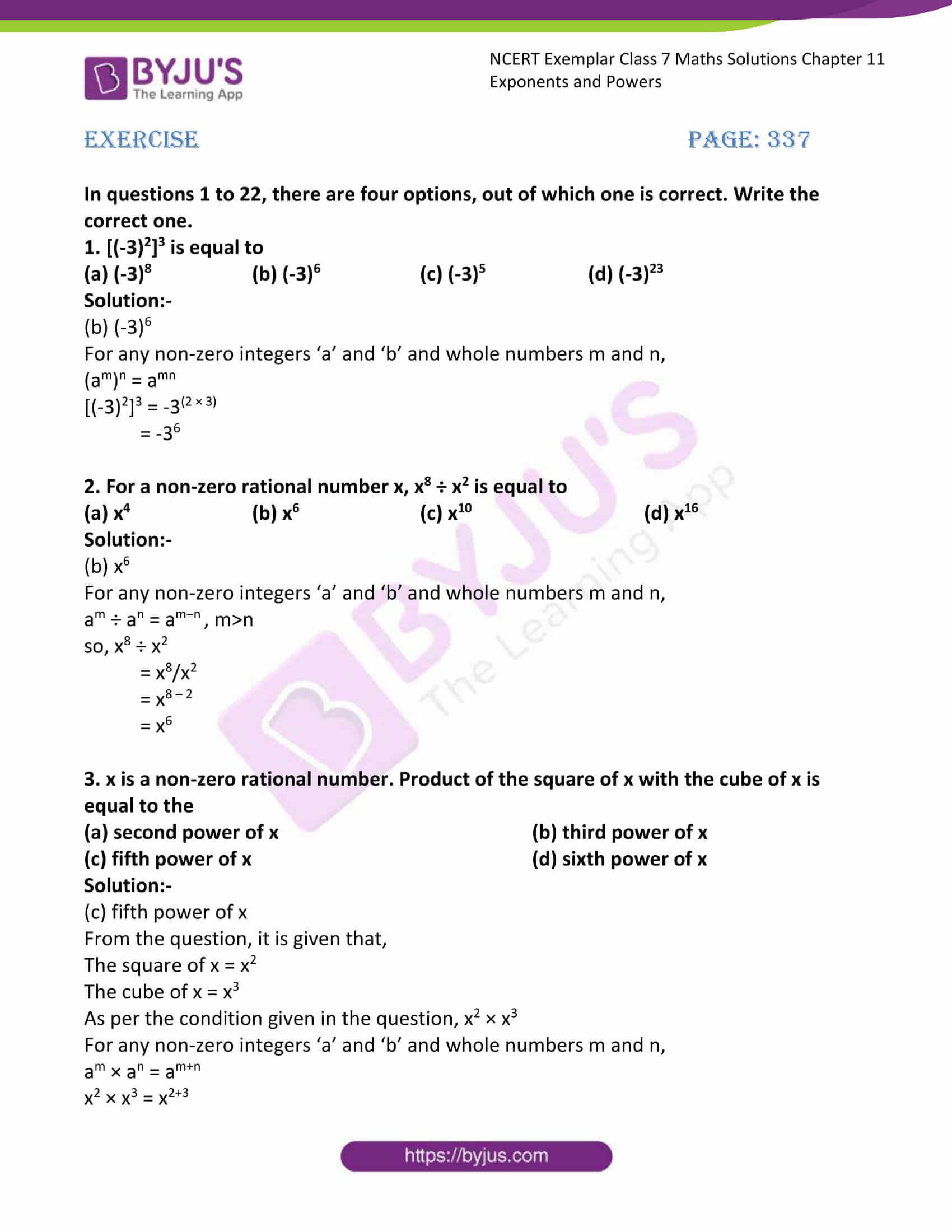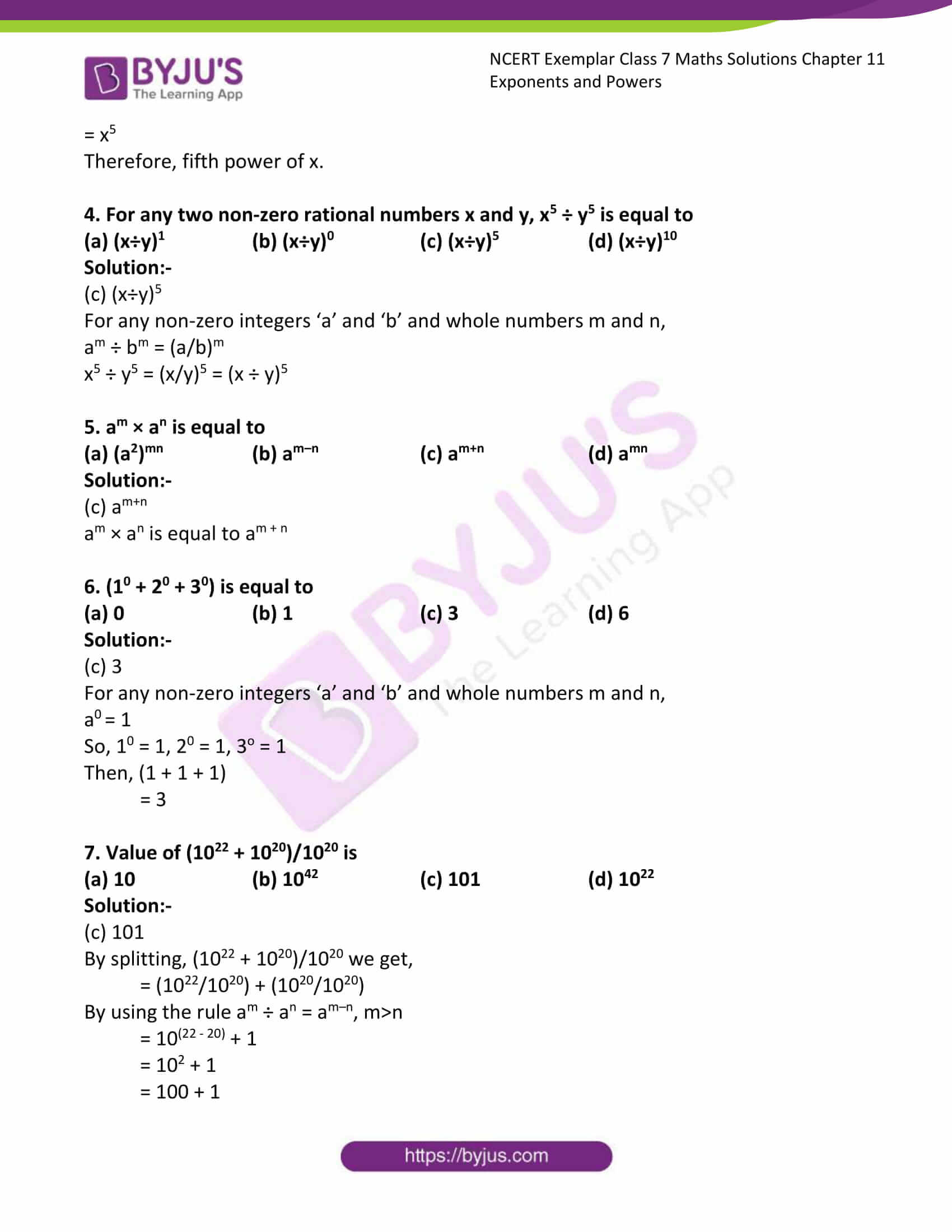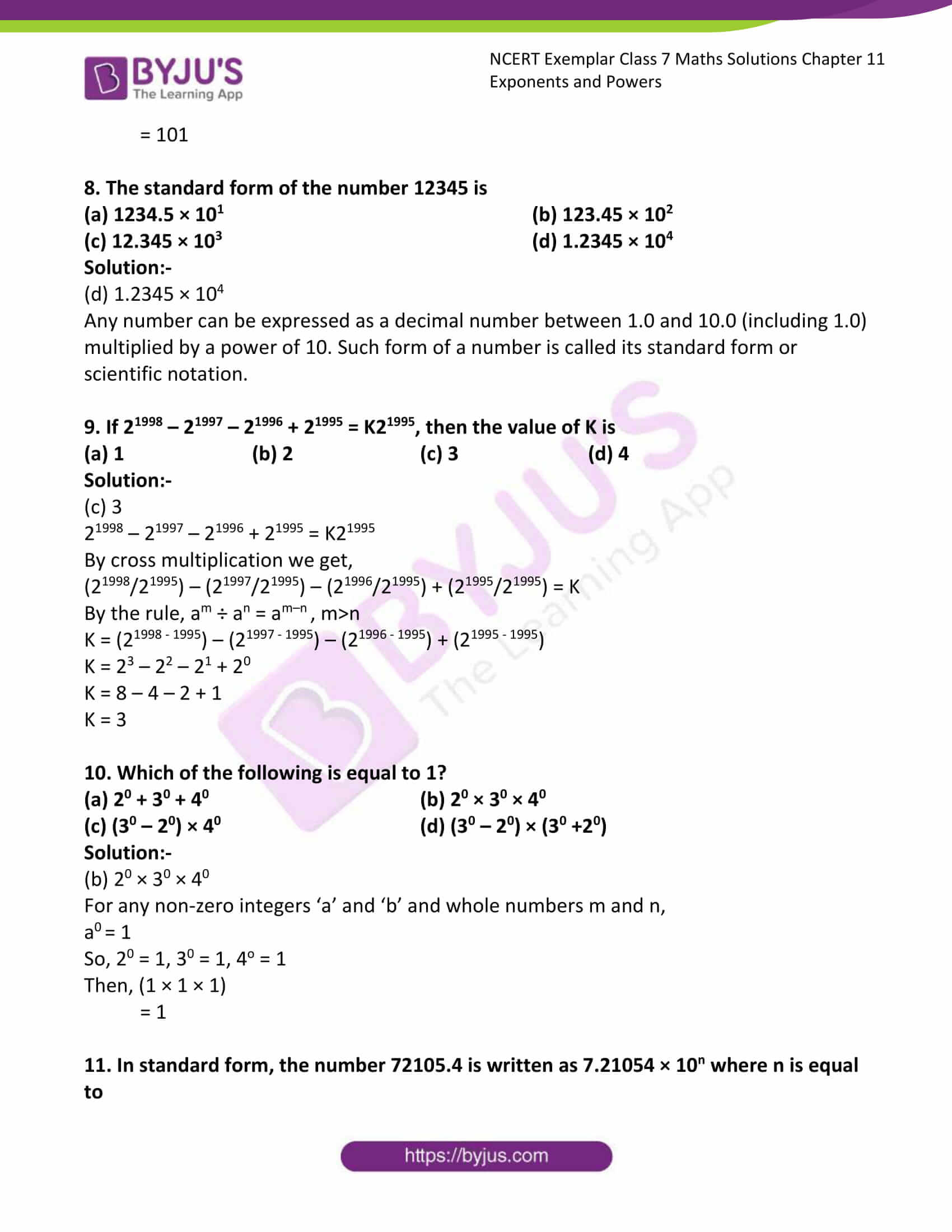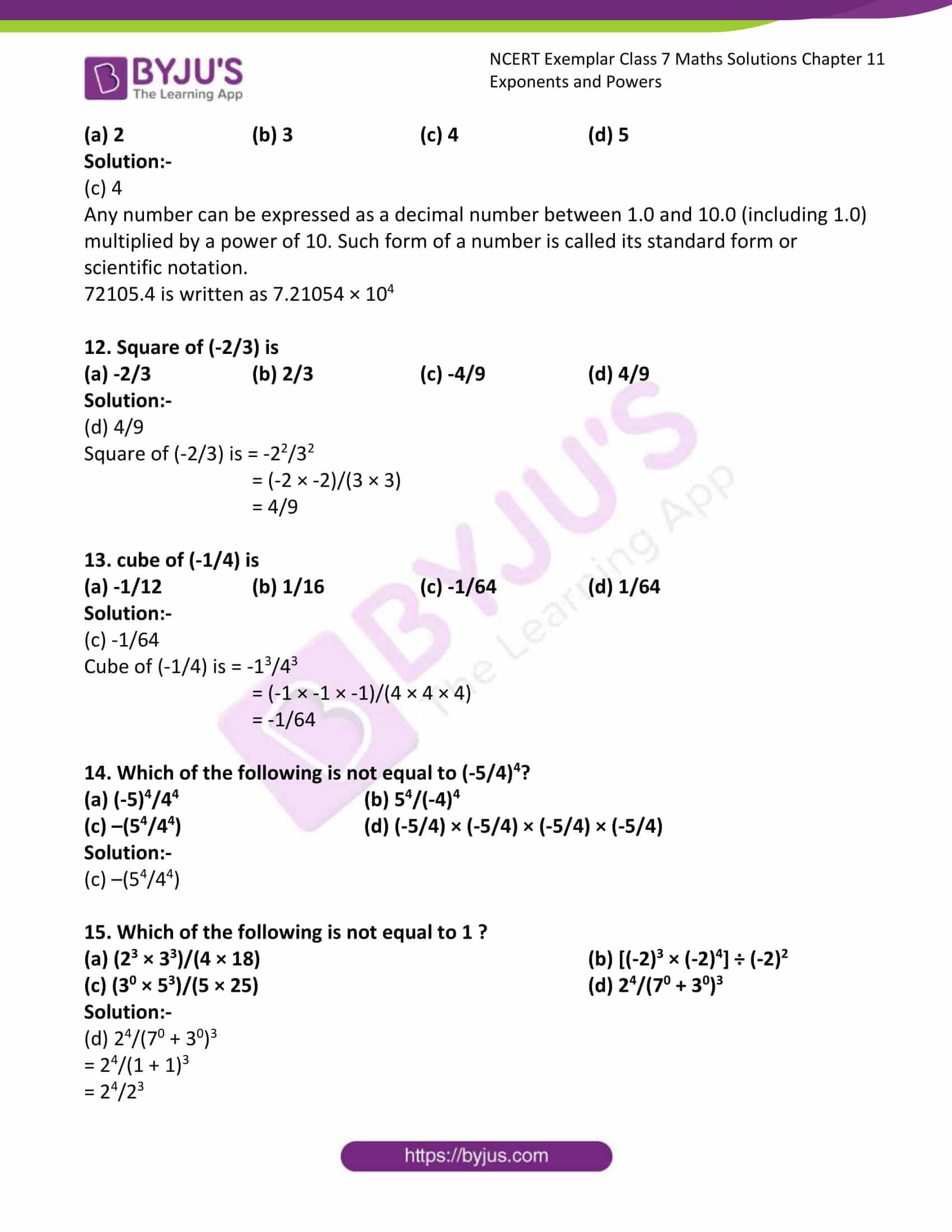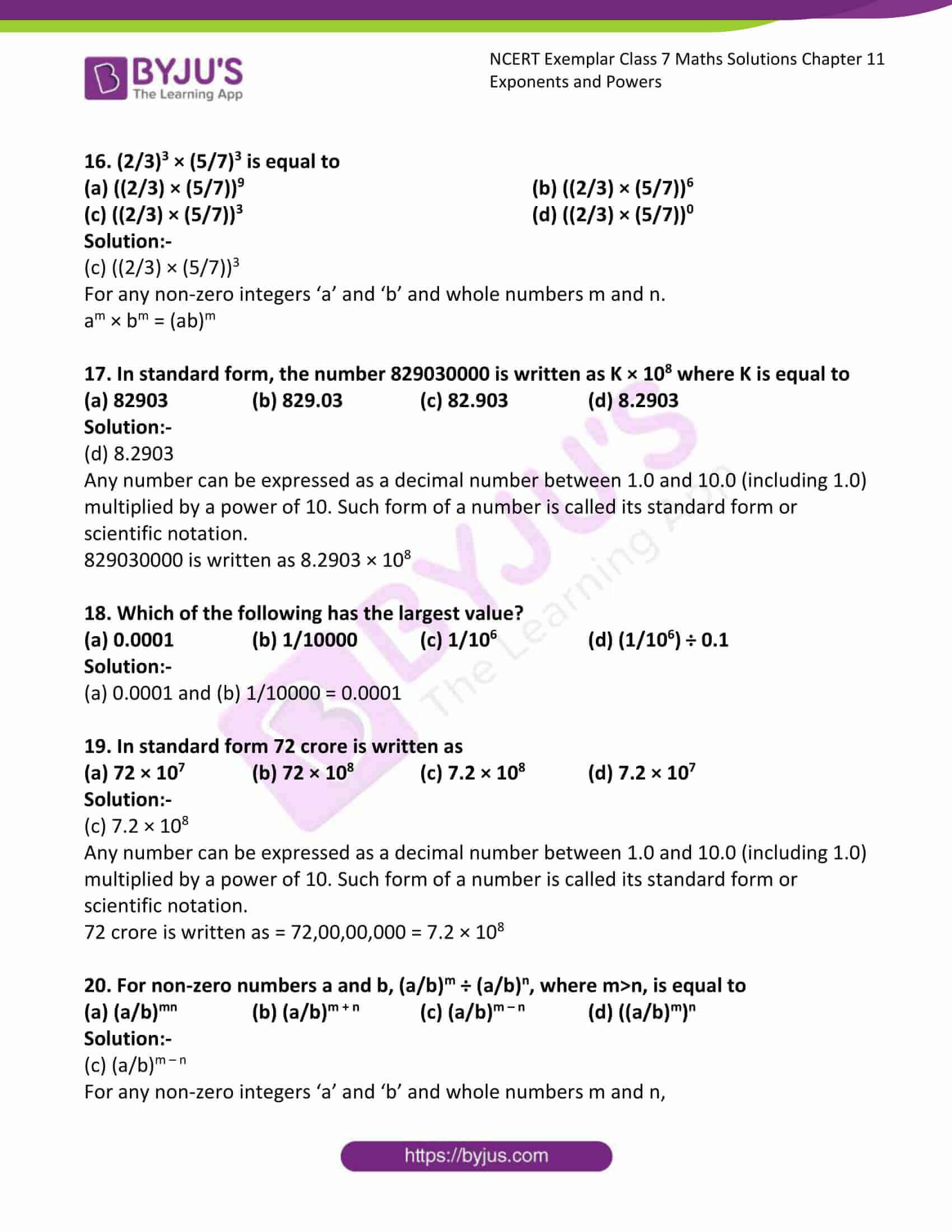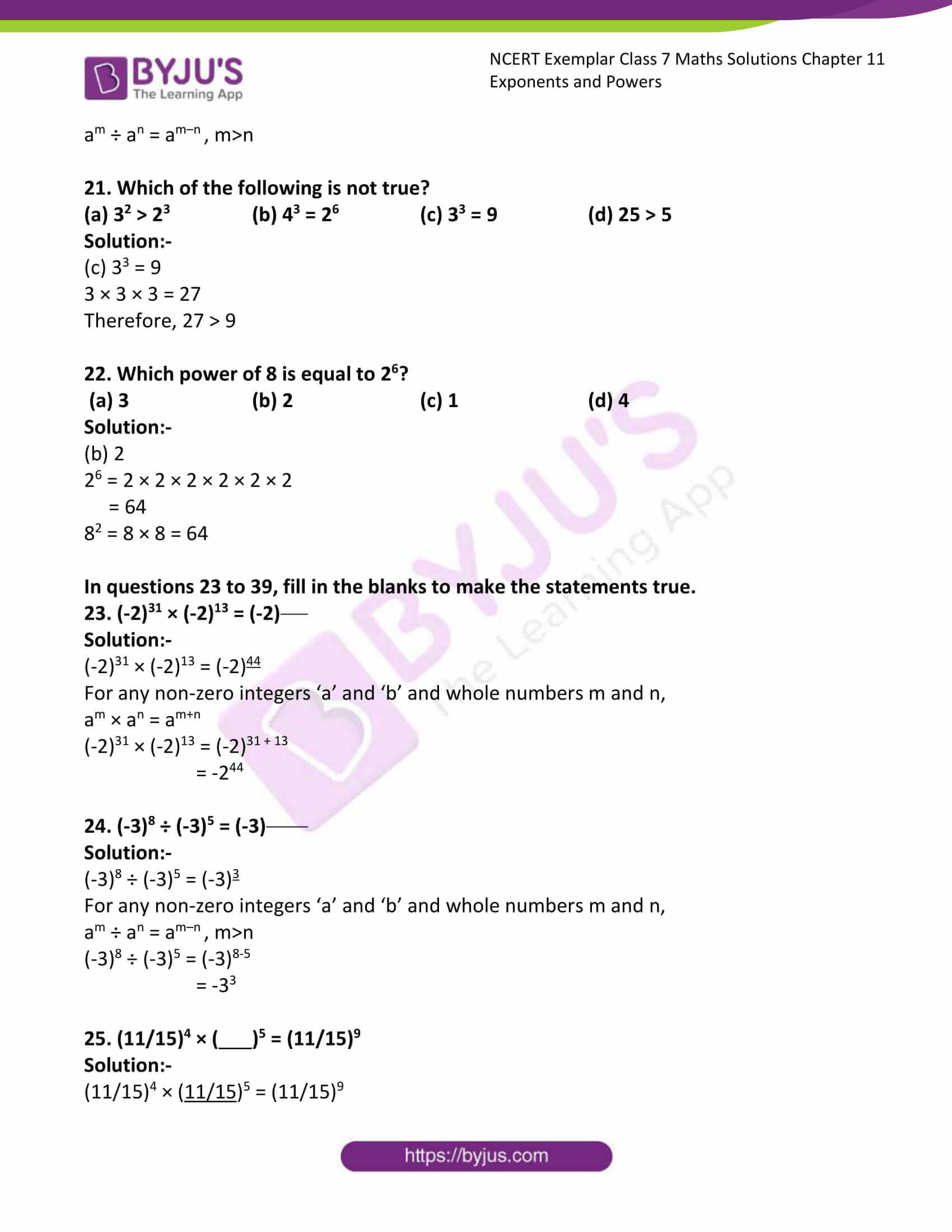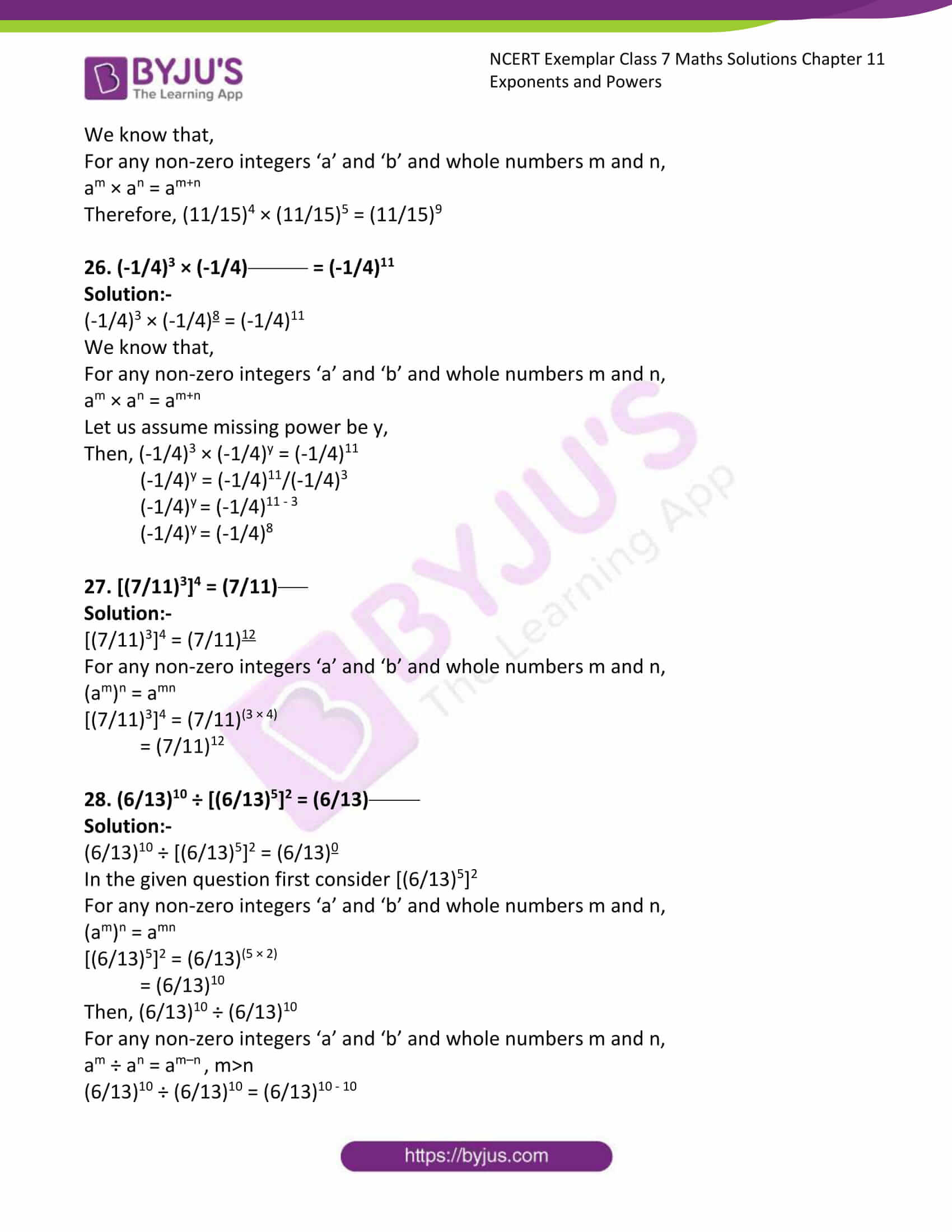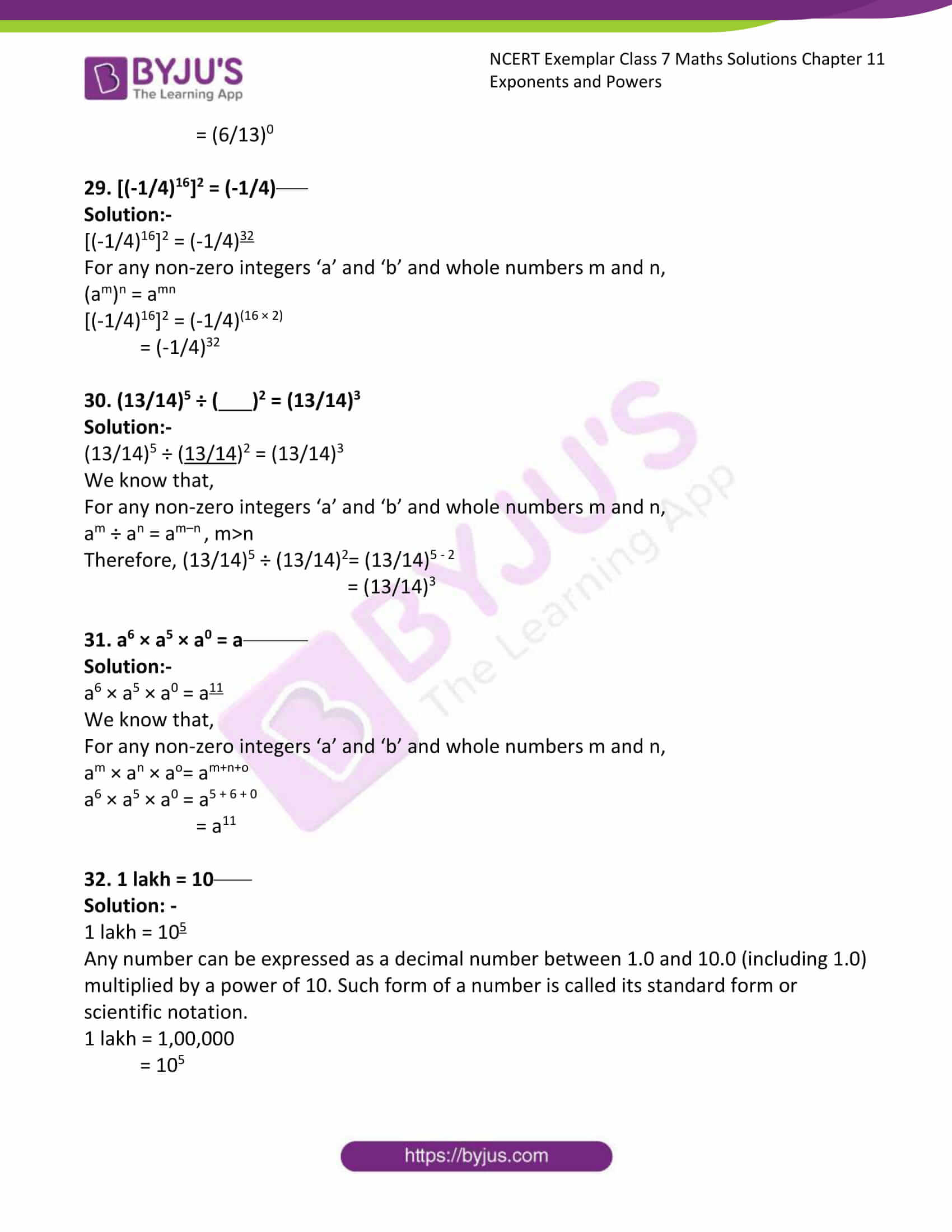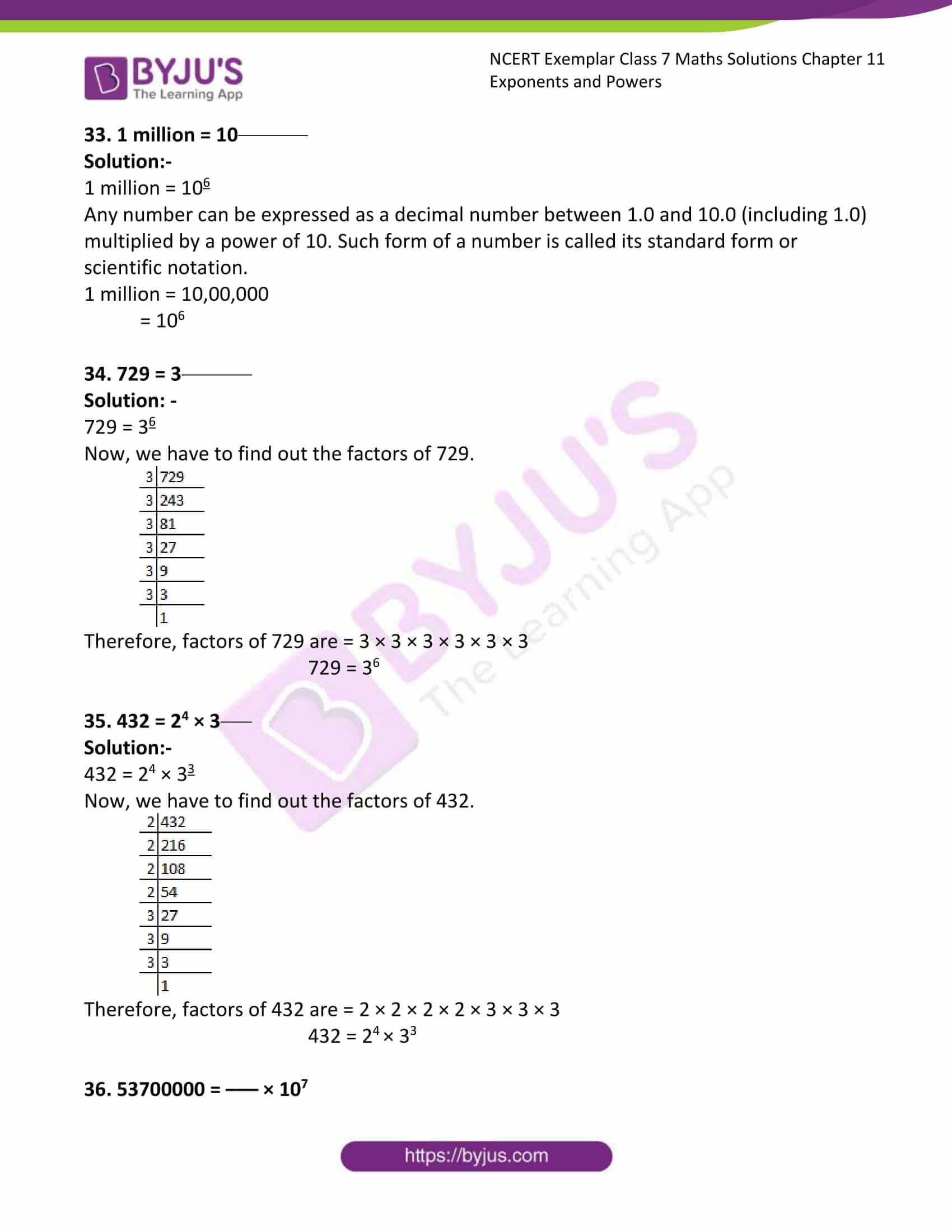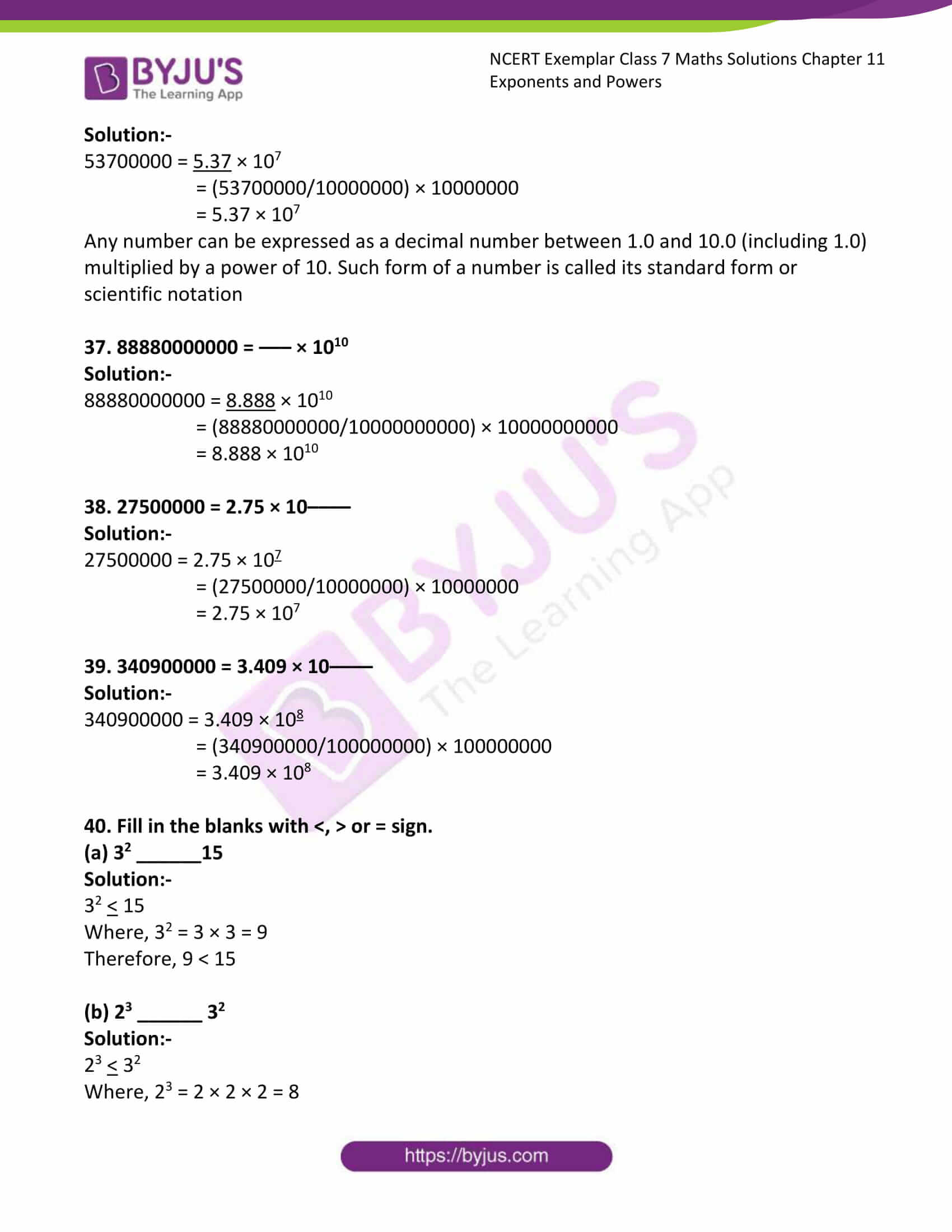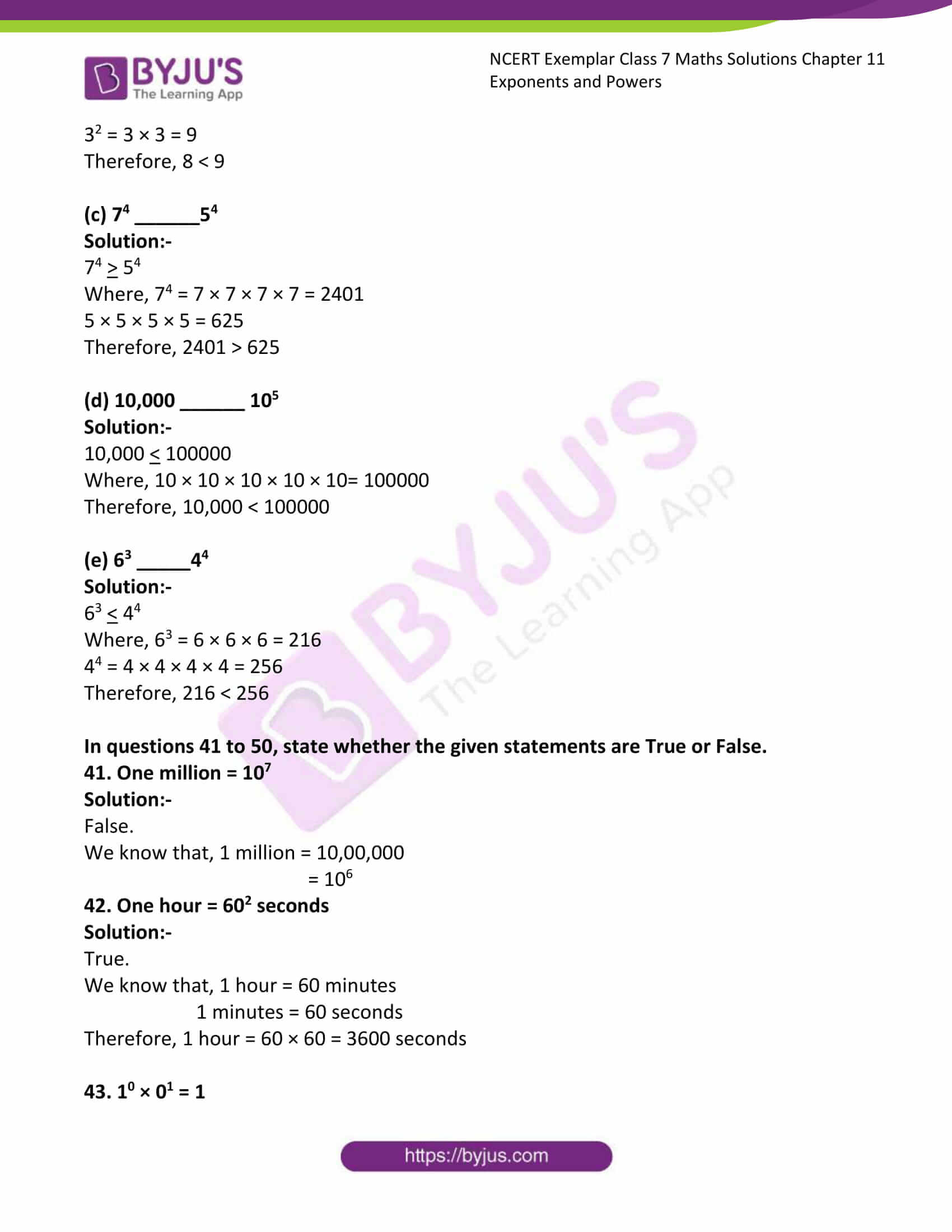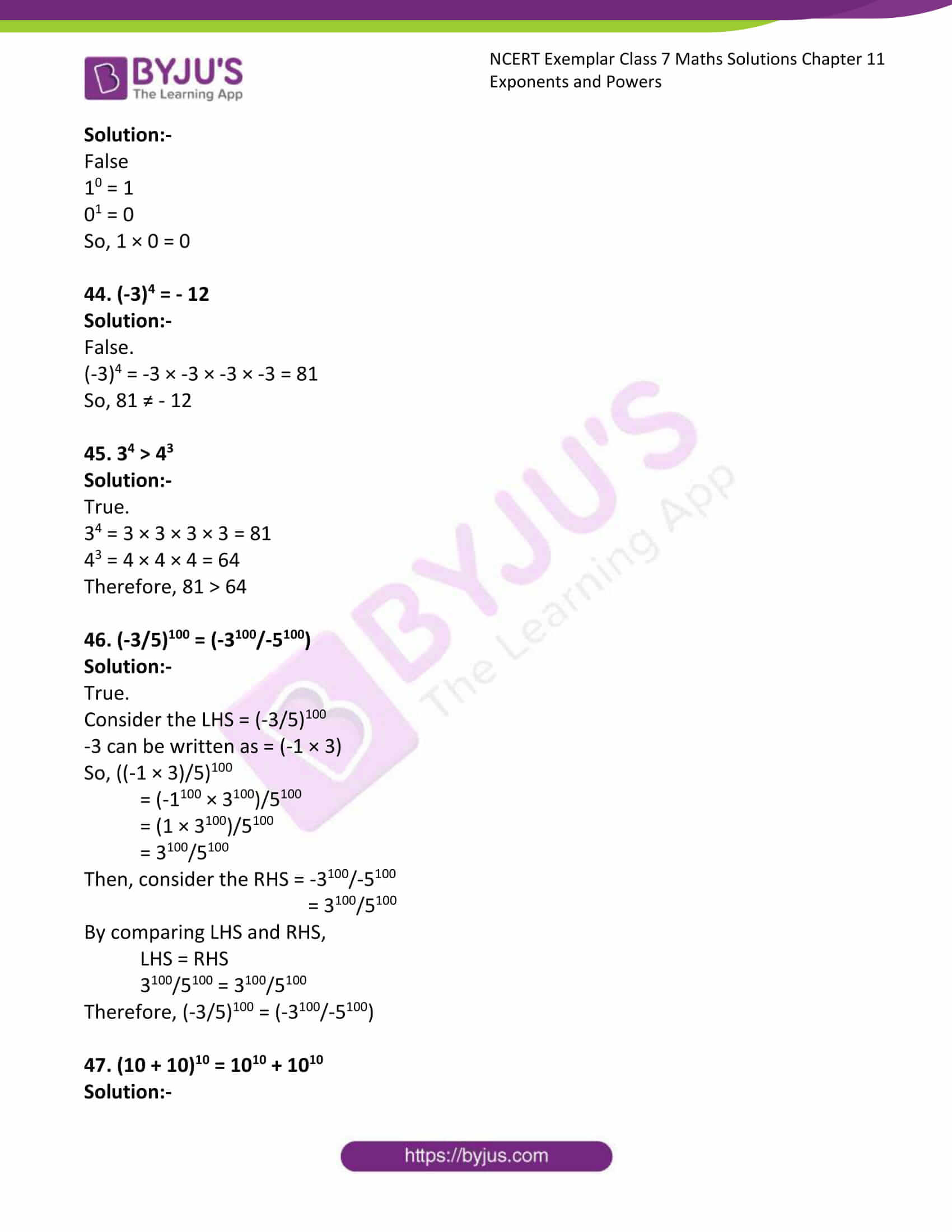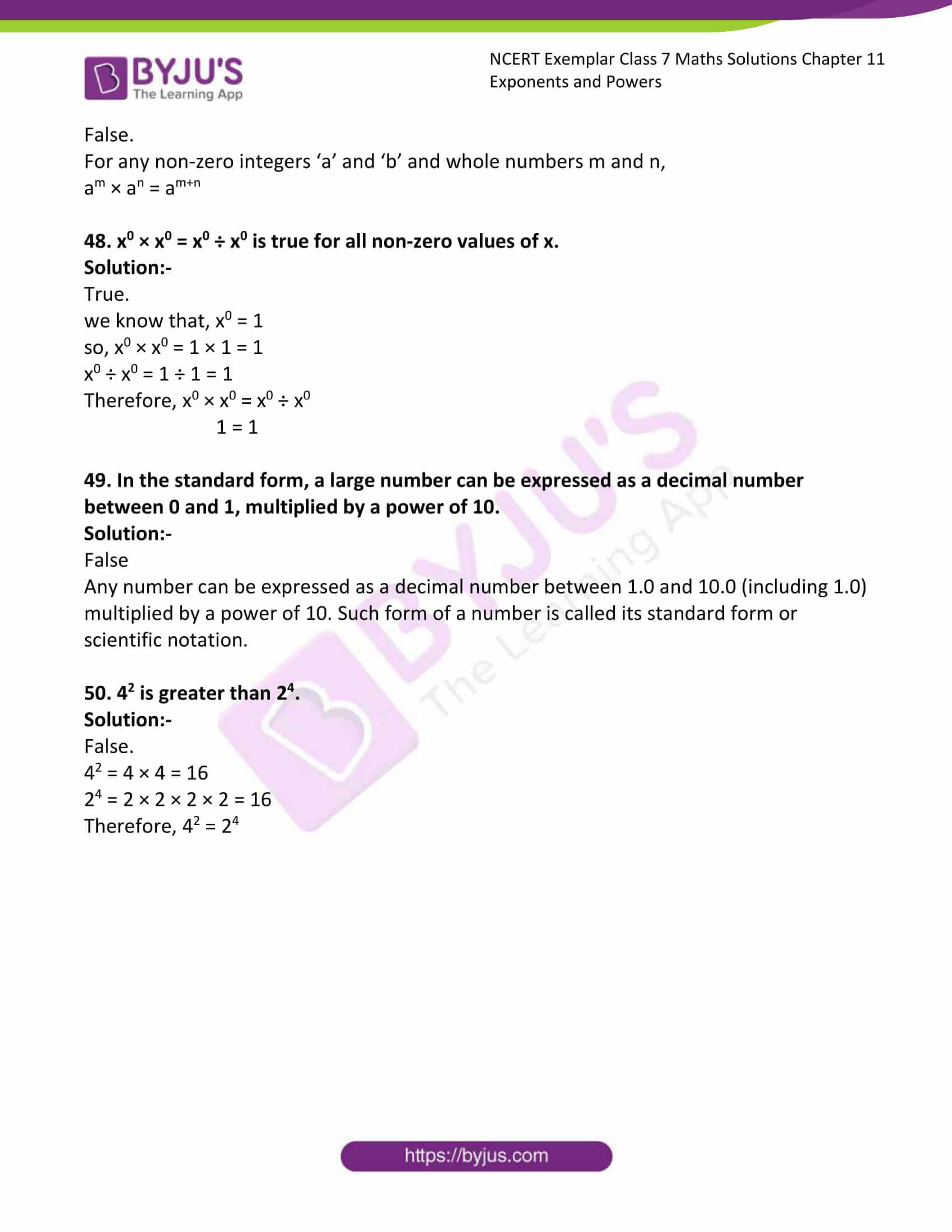### Access answers to Maths NCERT Exemplar Solutions for Class 7 Chapter 11 Exponents and Powers

Exercise Page: 337

In questions 1 to 22, there are four options, out of which one is correct. Write the correct one.

1. [(-3)2]3 is equal to

(a) (-3)8 (b) (-3)6 (c) (-3)5 (d) (-3)23

Solution:-

(b) (-3)6

For any non-zero integers ‘a’ and ‘b’ and whole numbers m and n,

(am)n = amn

[(-3)2]3 = -3(2 × 3)

= -36

2. For a non-zero rational number x, x8 ÷ x2 is equal to

(a) x4 (b) x6 (c) x10 (d) x16

Solution:-

(b) x6

For any non-zero integers ‘a’ and ‘b’ and whole numbers m and n,

am ÷ an = am–n , m>n

so, x8 ÷ x2

= x8/x2

= x8 – 2

= x6

3. x is a non-zero rational number. Product of the square of x with the cube of x is equal to the

(a) second power of x (b) third power of x

(c) fifth power of x (d) sixth power of x

Solution:-

(c) fifth power of x

From the question, it is given that,

The square of x = x2

The cube of x = x3

As per the condition given in the question, x2 × x3

For any non-zero integers ‘a’ and ‘b’ and whole numbers m and n,

am × an = am+n

x2 × x3 = x2+3

= x5

Therefore, fifth power of x.

4. For any two non-zero rational numbers x and y, x5 ÷ y5 is equal to

(a) (x÷y)1 (b) (x÷y)0 (c) (x÷y)5 (d) (x÷y)10

Solution:-

(c) (x÷y)5

For any non-zero integers ‘a’ and ‘b’ and whole numbers m and n,

am ÷ bm = (a/b)m

x5 ÷ y5 = (x/y)5 = (x ÷ y)5

5. am × an is equal to

(a) (a2)mn (b) am–n (c) am+n (d) amn

Solution:-

(c) am+n

am × an is equal to am + n

6. (10 + 20 + 30) is equal to

(a) 0 (b) 1 (c) 3 (d) 6

Solution:-

(c) 3

For any non-zero integers ‘a’ and ‘b’ and whole numbers m and n,

a0 = 1

So, 10 = 1, 20 = 1, 3o = 1

Then, (1 + 1 + 1)

= 3

7. Value of (1022 + 1020)/1020 is

(a) 10 (b) 1042 (c) 101 (d) 1022

Solution:-

(c) 101

By splitting, (1022 + 1020)/1020 we get,

= (1022/1020) + (1020/1020)

By using the rule am ÷ an = am–n, m>n

= 10(22 – 20) + 1

= 102 + 1

= 100 + 1

= 101

8. The standard form of the number 12345 is

(a) 1234.5 × 101 (b) 123.45 × 102

(c) 12.345 × 103 (d) 1.2345 × 104

Solution:-

(d) 1.2345 × 104

Any number can be expressed as a decimal number between 1.0 and 10.0 (including 1.0) multiplied by a power of 10. Such form of a number is called its standard form or scientific notation.

9. If 21998 – 21997 – 21996 + 21995 = K21995, then the value of K is

(a) 1 (b) 2 (c) 3 (d) 4

Solution:-

(c) 3

21998 – 21997 – 21996 + 21995 = K21995

By cross multiplication we get,

(21998/21995) – (21997/21995) – (21996/21995) + (21995/21995) = K

By the rule, am ÷ an = am–n , m>n

K = (21998 – 1995) – (21997 – 1995) – (21996 – 1995) + (21995 – 1995)

K = 23 – 22 – 21 + 20

K = 8 – 4 – 2 + 1

K = 3

10. Which of the following is equal to 1?

(a) 20 + 30 + 40 (b) 20 × 30 × 40

(c) (30 – 20) × 40 (d) (30 – 20) × (30 +20)

Solution:-

(b) 20 × 30 × 40

For any non-zero integers ‘a’ and ‘b’ and whole numbers m and n,

a0 = 1

So, 20 = 1, 30 = 1, 4o = 1

Then, (1 × 1 × 1)

= 1

11. In standard form, the number 72105.4 is written as 7.21054 × 10n where n is equal to

(a) 2 (b) 3 (c) 4 (d) 5

Solution:-

(c) 4

Any number can be expressed as a decimal number between 1.0 and 10.0 (including 1.0) multiplied by a power of 10. Such form of a number is called its standard form or scientific notation.

72105.4 is written as 7.21054 × 104

12. Square of (-2/3) is

(a) -2/3 (b) 2/3 (c) -4/9 (d) 4/9

Solution:-

(d) 4/9

Square of (-2/3) is = -22/32

= (-2 × -2)/(3 × 3)

= 4/9

13. cube of (-1/4) is

(a) -1/12 (b) 1/16 (c) -1/64 (d) 1/64

Solution:-

(c) -1/64

Cube of (-1/4) is = -13/43

= (-1 × -1 × -1)/(4 × 4 × 4)

= -1/64

14. Which of the following is not equal to (-5/4)4?

(a) (-5)4/44 (b) 54/(-4)4

(c) –(54/44) (d) (-5/4) × (-5/4) × (-5/4) × (-5/4)

Solution:-

(c) –(54/44)

15. Which of the following is not equal to 1 ?

(a) (23 × 33)/(4 × 18) (b) [(-2)3 × (-2)4] ÷ (-2)2

(c) (30 × 53)/(5 × 25) (d) 24/(70 + 30)3

Solution:-

(d) 24/(70 + 30)3

= 24/(1 + 1)3

= 24/23

16. (2/3)3 × (5/7)3 is equal to

(a) ((2/3) × (5/7))9 (b) ((2/3) × (5/7))6

(c) ((2/3) × (5/7))3 (d) ((2/3) × (5/7))0

Solution:-

(c) ((2/3) × (5/7))3

For any non-zero integers ‘a’ and ‘b’ and whole numbers m and n.

am × bm = (ab)m

17. In standard form, the number 829030000 is written as K × 108 where K is equal to (a) 82903 (b) 829.03 (c) 82.903 (d) 8.2903

Solution:-

(d) 8.2903

Any number can be expressed as a decimal number between 1.0 and 10.0 (including 1.0) multiplied by a power of 10. Such form of a number is called its standard form or scientific notation.

829030000 is written as 8.2903 × 108

18. Which of the following has the largest value?

(a) 0.0001 (b) 1/10000 (c) 1/106 (d) (1/106) ÷ 0.1

Solution:-

(a) 0.0001 and (b) 1/10000 = 0.0001

19. In standard form 72 crore is written as

(a) 72 × 107 (b) 72 × 108 (c) 7.2 × 108 (d) 7.2 × 107

Solution:-

(c) 7.2 × 108

Any number can be expressed as a decimal number between 1.0 and 10.0 (including 1.0) multiplied by a power of 10. Such form of a number is called its standard form or scientific notation.

72 crore is written as = 72,00,00,000 = 7.2 × 108

20. For non-zero numbers a and b, (a/b)m ÷ (a/b)n, where m>n, is equal to

(a) (a/b)mn (b) (a/b)m + n (c) (a/b)m – n (d) ((a/b)m)n

Solution:-

(c) (a/b)m – n

For any non-zero integers ‘a’ and ‘b’ and whole numbers m and n,

am ÷ an = am–n , m>n

21. Which of the following is not true?

(a) 32 > 23 (b) 43 = 26 (c) 33 = 9 (d) 25 > 5

Solution:-

(c) 33 = 9

3 × 3 × 3 = 27

Therefore, 27 > 9

22. Which power of 8 is equal to 26?

(a) 3 (b) 2 (c) 1 (d) 4

Solution:-

(b) 2

26 = 2 × 2 × 2 × 2 × 2 × 2

= 64

82 = 8 × 8 = 64

In questions 23 to 39, fill in the blanks to make the statements true.

23. (-2)31 × (-2)13 = (-2)

Solution:-

(-2)31 × (-2)13 = (-2)44

For any non-zero integers ‘a’ and ‘b’ and whole numbers m and n,

am × an = am+n

(-2)31 × (-2)13 = (-2)31 + 13

= -244

24. (-3)8 ÷ (-3)5 = (-3)

Solution:-

(-3)8 ÷ (-3)5 = (-3)3

For any non-zero integers ‘a’ and ‘b’ and whole numbers m and n,

am ÷ an = am–n , m>n

(-3)8 ÷ (-3)5 = (-3)8-5

= -33

25. (11/15)4 × ( )5 = (11/15)9

Solution:-

(11/15)4 × (11/15)5 = (11/15)9

We know that,

For any non-zero integers ‘a’ and ‘b’ and whole numbers m and n,

am × an = am+n

Therefore, (11/15)4 × (11/15)5 = (11/15)9

26. (-1/4)3 × (-1/4) = (-1/4)11

Solution:-

(-1/4)3 × (-1/4)8 = (-1/4)11

We know that,

For any non-zero integers ‘a’ and ‘b’ and whole numbers m and n,

am × an = am+n

Let us assume missing power be y,

Then, (-1/4)3 × (-1/4)y = (-1/4)11

(-1/4)y = (-1/4)11/(-1/4)3

(-1/4)y = (-1/4)11 – 3

(-1/4)y = (-1/4)8

27. [(7/11)3]4 = (7/11)

Solution:-

[(7/11)3]4 = (7/11)12

For any non-zero integers ‘a’ and ‘b’ and whole numbers m and n,

(am)n = amn

[(7/11)3]4 = (7/11)(3 × 4)

= (7/11)12

28. (6/13)10 ÷ [(6/13)5]2 = (6/13)

Solution:-

(6/13)10 ÷ [(6/13)5]2 = (6/13)0

In the given question first consider [(6/13)5]2

For any non-zero integers ‘a’ and ‘b’ and whole numbers m and n,

(am)n = amn

[(6/13)5]2 = (6/13)(5 × 2)

= (6/13)10

Then, (6/13)10 ÷ (6/13)10

For any non-zero integers ‘a’ and ‘b’ and whole numbers m and n,

am ÷ an = am–n , m>n

(6/13)10 ÷ (6/13)10 = (6/13)10 – 10

= (6/13)0

29. [(-1/4)16]2 = (-1/4)

Solution:-

[(-1/4)16]2 = (-1/4)32

For any non-zero integers ‘a’ and ‘b’ and whole numbers m and n,

(am)n = amn

[(-1/4)16]2 = (-1/4)(16 × 2)

= (-1/4)32

30. (13/14)5 ÷ ( )2 = (13/14)3

Solution:-

(13/14)5 ÷ (13/14)2 = (13/14)3

We know that,

For any non-zero integers ‘a’ and ‘b’ and whole numbers m and n,

am ÷ an = am–n , m>n

Therefore, (13/14)5 ÷ (13/14)2= (13/14)5 – 2

= (13/14)3

31. a6 × a5 × a0 = a

Solution:-

a6 × a5 × a0 = a11

We know that,

For any non-zero integers ‘a’ and ‘b’ and whole numbers m and n,

am × an × ao= am+n+o

a6 × a5 × a0 = a5 + 6 + 0

= a11

32. 1 lakh = 10

Solution: –

1 lakh = 105

Any number can be expressed as a decimal number between 1.0 and 10.0 (including 1.0) multiplied by a power of 10. Such form of a number is called its standard form or scientific notation.

1 lakh = 1,00,000

= 105

33. 1 million = 10

Solution:-

1 million = 106

Any number can be expressed as a decimal number between 1.0 and 10.0 (including 1.0) multiplied by a power of 10. Such form of a number is called its standard form or scientific notation.

1 million = 10,00,000

= 106

34. 729 = 3

Solution: –

729 = 36

Now, we have to find out the factors of 729.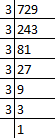Therefore, factors of 729 are = 3 × 3 × 3 × 3 × 3 × 3

729 = 36

35. 432 = 24 × 3

Solution:-

432 = 24 × 33

Now, we have to find out the factors of 432.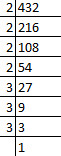Therefore, factors of 432 are = 2 × 2 × 2 × 2 × 3 × 3 × 3

432 = 24 × 33

36. 53700000 = ––– × 107

Solution:-

53700000 = 5.37 × 107

= (53700000/10000000) × 10000000

= 5.37 × 107

Any number can be expressed as a decimal number between 1.0 and 10.0 (including 1.0) multiplied by a power of 10. Such form of a number is called its standard form or scientific notation

37. 88880000000 = ––– × 1010

Solution:-

88880000000 = 8.888 × 1010

= (88880000000/10000000000) × 10000000000

= 8.888 × 1010

38. 27500000 = 2.75 × 10––––

Solution:-

27500000 = 2.75 × 107

= (27500000/10000000) × 10000000

= 2.75 × 107

39. 340900000 = 3.409 × 10––––

Solution:-

340900000 = 3.409 × 108

= (340900000/100000000) × 100000000

= 3.409 × 108

40. Fill in the blanks with <, > or = sign.

(a) 32 ______15

Solution:-

32 < 15

Where, 32 = 3 × 3 = 9

Therefore, 9 < 15

(b) 23 ______ 32

Solution:-

23 < 32

Where, 23 = 2 × 2 × 2 = 8

32 = 3 × 3 = 9

Therefore, 8 < 9

(c) 74 ______54

Solution:-

74 > 54

Where, 74 = 7 × 7 × 7 × 7 = 2401

5 × 5 × 5 × 5 = 625

Therefore, 2401 > 625

(d) 10,000 ______ 105

Solution:-

10,000 < 100000

Where, 10 × 10 × 10 × 10 × 10= 100000

Therefore, 10,000 < 100000

(e) 63 _____44

Solution:-

63 < 44

Where, 63 = 6 × 6 × 6 = 216

44 = 4 × 4 × 4 × 4 = 256

Therefore, 216 < 256

In questions 41 to 50, state whether the given statements are True or False.

41. One million = 107

Solution:-

False.

We know that, 1 million = 10,00,000

= 106

42. One hour = 602 seconds

Solution:-

True.

We know that, 1 hour = 60 minutes

1 minutes = 60 seconds

Therefore, 1 hour = 60 × 60 = 3600 seconds

43. 10 × 01 = 1

Solution:-

False

10 = 1

01 = 0

So, 1 × 0 = 0

44. (-3)4 = – 12

Solution:-

False.

(-3)4 = -3 × -3 × -3 × -3 = 81

So, 81 ≠ – 12

45. 34 > 43

Solution:-

True.

34 = 3 × 3 × 3 × 3 = 81

43 = 4 × 4 × 4 = 64

Therefore, 81 > 64

46. (-3/5)100 = (-3100/-5100)

Solution:-

True.

Consider the LHS = (-3/5)100

-3 can be written as = (-1 × 3)

So, ((-1 × 3)/5)100

= (-1100 × 3100)/5100

= (1 × 3100)/5100

= 3100/5100

Then, consider the RHS = -3100/-5100

= 3100/5100

By comparing LHS and RHS,

LHS = RHS

3100/5100 = 3100/5100

Therefore, (-3/5)100 = (-3100/-5100)

47. (10 + 10)10 = 1010 + 1010

Solution:-

False.

For any non-zero integers ‘a’ and ‘b’ and whole numbers m and n,

am × an = am+n

48. x0 × x0 = x0 ÷ x0 is true for all non-zero values of x.

Solution:-

True.

we know that, x0 = 1

so, x0 × x0 = 1 × 1 = 1

x0 ÷ x0 = 1 ÷ 1 = 1

Therefore, x0 × x0 = x0 ÷ x0

1 = 1

49. In the standard form, a large number can be expressed as a decimal number between 0 and 1, multiplied by a power of 10.

Solution:-

False

Any number can be expressed as a decimal number between 1.0 and 10.0 (including 1.0) multiplied by a power of 10. Such form of a number is called its standard form or scientific notation.

50. 42 is greater than 24.

Solution:-

False.

42 = 4 × 4 = 16

24 = 2 × 2 × 2 × 2 = 16

Therefore, 42 = 24